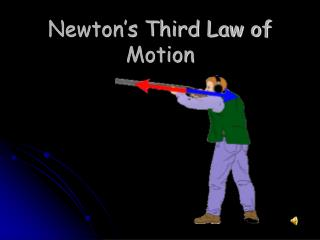DownloadDownload PresentationNewton’s Third Law of Motion

# Newton’s Third Law of Motion

Download Presentation## Newton’s Third Law of Motion

- - - - - - - - - - - - - - - - - - - - - - - - - - - E N D - - - - - - - - - - - - - - - - - - - - - - - - - - -
##### Presentation Transcript

1. Newton’s Third Law of Motion

2. For every force there is an equal force in the opposite direction. http://teachertube.com/viewVideo.php?video_id=21646

3. This is often stated as the “Law of Pairs” because all forces come in pairs. “For every action, there is an equal and opposite reaction.” THOSE ARE PEARS!!!

4. When you sit down on your chair you are applying a downward force on the chair. If the chair does not apply an upward force that is equal to the force you are pushing down, where will you be sitting? • ON THE FLOOR!

5. How does a rocket take off? Fuel is burned inside the engine producing hot gases. The gases are pushed down and out of the rocket. As the gases move downward, the rocket moves in the opposite direction, upwards.

6. Momentum = mass * velocity p = m * v

7. Momentum • P = m x v • P = Momentum (kg x m/s) • m = Mass (kg) • V = speed (m/s)

8. 1. While driving down the road, Anna observed a bug striking the windshield of her car. Quite obviously, a case of Newton's third law of motion. The bug hit the windshield and the windshield hit the bug. Which of the two forces is greater: the force on the bug or the force on the windshield?

9. Trick question! Each force is the same size. • For every action there is an equal and opposite reaction. • The fact that the bug splatters only means that with its smaller mass it is less able to withstand the larger acceleration resulting from the interaction.

10. 2. A gun recoils when it is fired. The recoil is the result of action-reaction force pairs. As the gases from the gunpowder explosion expand, the gun pushes the bullet forwards and the bullet pushes the gun backwards. The acceleration of the recoiling gun is ...

11. A. greater than the acceleration of the bullet. • B. smaller than the acceleration of the bullet. • C. the same size as the acceleration of the bullet.

12. B. smaller than the acceleration of the bullet. • The force on the gun equals the force on the bullet. • The bullet b/c it has a smaller mass and so has a greater acceleration.